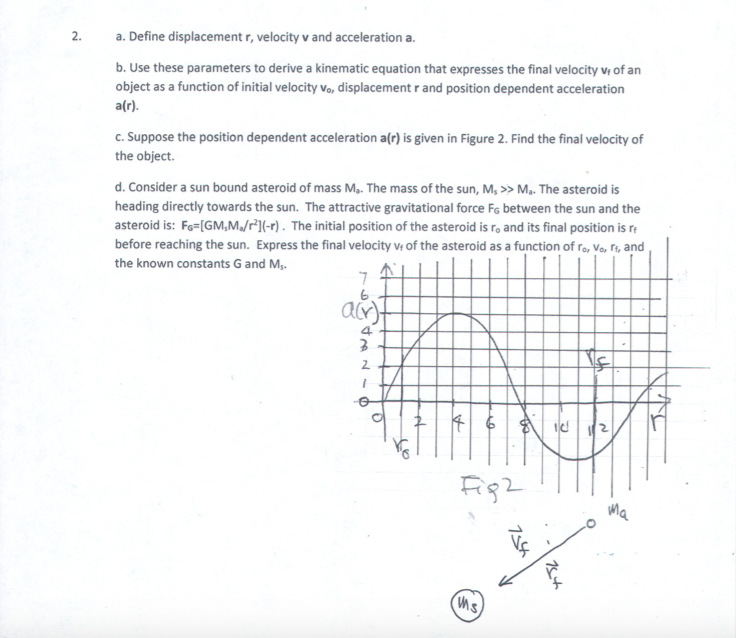# 2. a. Define displacement r, velocity v and acceleration a b. Use these parameters to derive a kinematic equation that expresses the final velocity vi of an object as a function of nitial velocity Vo, displacement r and position dependent acceleration a(r). C.Suppose the position dependent acceleration a(r) is given in Figure 2. Find the final velocity of the object d. Consider a sun bound asteroid of mass Ma. The mass of the sun, M,>>Ma. The asteroid is heading directly towards the sun. The attractive gravitational force Fs between the sun and the asteroid is: Fo IGM,M/r1l-r). The initial position of the asteroid is ro and its final position is r before reaching the sun. Express the final velocity of the asteroid as a function of ro, Vo, ri, and the known constants G and M 6 4. 2. 2

Questionhelp_outlineImage Transcriptionclose2. a. Define displacement r, velocity v and acceleration a b. Use these parameters to derive a kinematic equation that expresses the final velocity vi of an object as a function of nitial velocity Vo, displacement r and position dependent acceleration a(r). C.Suppose the position dependent acceleration a(r) is given in Figure 2. Find the final velocity of the object d. Consider a sun bound asteroid of mass Ma. The mass of the sun, M,>>Ma. The asteroid is heading directly towards the sun. The attractive gravitational force Fs between the sun and the asteroid is: Fo IGM,M/r1l-r). The initial position of the asteroid is ro and its final position is r before reaching the sun. Express the final velocity of the asteroid as a function of ro, Vo, ri, and the known constants G and M 6 4. 2. 2 fullscreen

## Expert Answer

### Want to see this answer and more?

Experts are waiting 24/7 to provide step-by-step solutions in as fast as 30 minutes!*

*Response times may vary by subject and question complexity. Median response time is 34 minutes for paid subscribers and may be longer for promotional offers.
Tagged in
Science
Physics

### Kinematics© 2021 bartleby. All Rights Reserved.